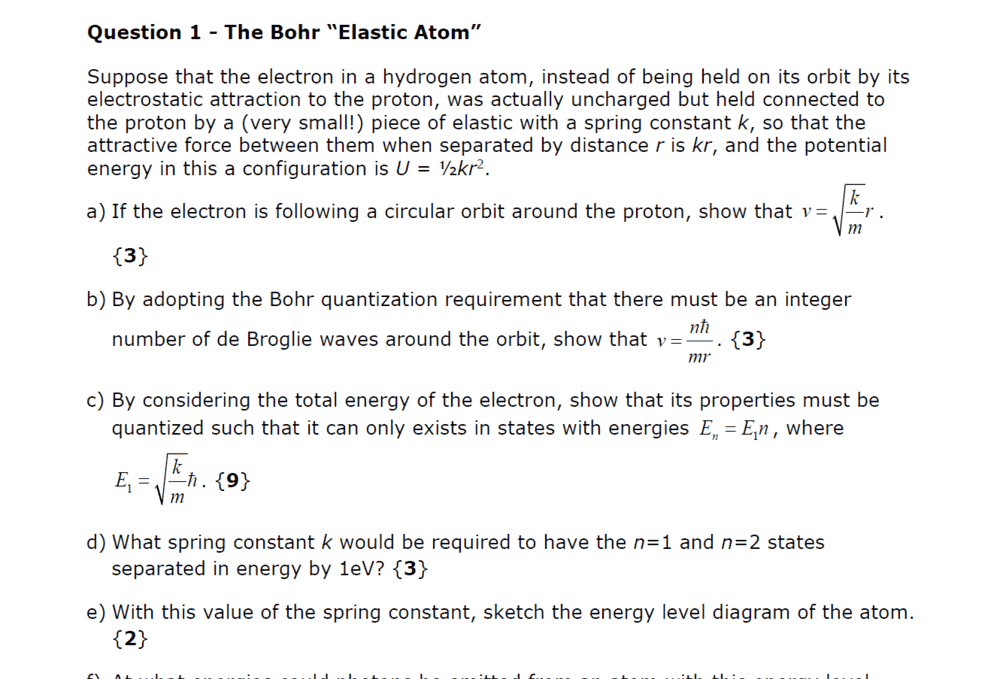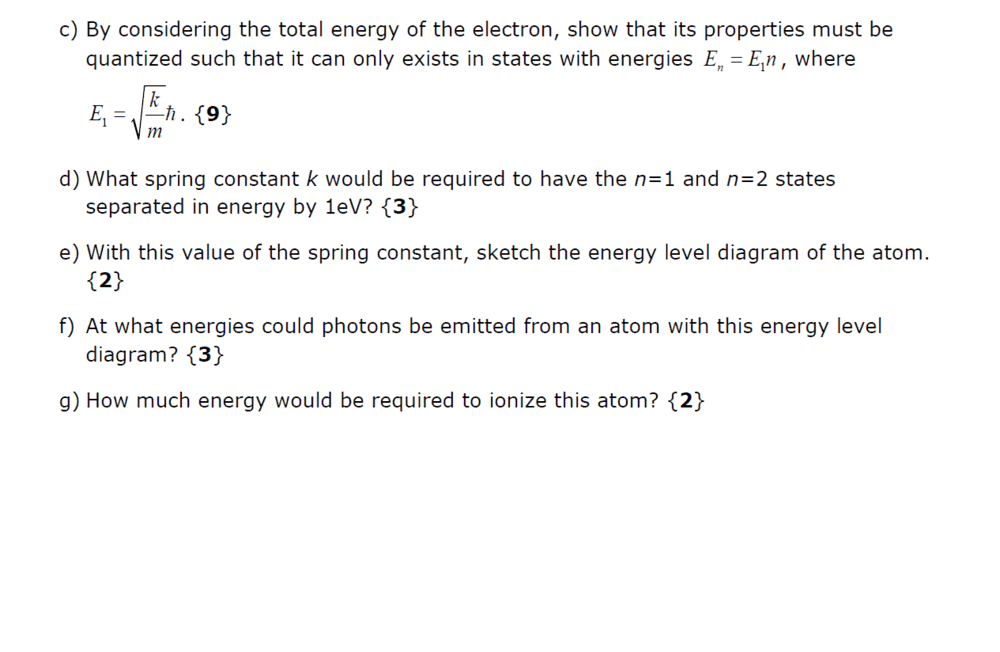# Bohr atom question

StillAnotherDave
Homework Statement:
Help with parts (c) and (e)
Relevant Equations:
Given in the screenshotHello folks,

I've managed questions (a) and (b) but don't get what to do with part (c). Normally you would equate the velocity equations v2=e2/4πεmr=(n2h2)/(m2r2 ). This let's you isolate the radius and use it to calculate En. But I can't see how you could do this for v given in (a) and (b).

Also, part (e). If En = E1n then the energy levels simply scale linearly ... that can't be right?

Last edited:

StillAnotherDave
Okay. I managed to solve part (c) by isolating r2.

But part (e) is still unclear ...

Homework Helper
Okay. I managed to solve part (c) by isolating r2.

But part (e) is still unclear ...
Remember, this is not a "real"atom where electric force acts between the proton and electron. You derived that En = Ein .Are those energy levels equidistant?

StillAnotherDave
They would be linear (equidistant) and positive - i.e. n=1 is 1eV; n=2 is 2eV; n=3 is 3eV ... is that correct?

But wouldn't n = ∞ need to be 0eV?

It makes the remaining parts of the question (f) and (g) very confusing:Last edited:
StillAnotherDave
Clearly this is ludicrous ... help!

Mentor
They would be linear (equidistant) and positive - i.e. n=1 is 1eV; n=2 is 2eV; n=3 is 3eV ... is that correct?
Yes.

But wouldn't n = ∞ need to be 0eV?
The choice of zero of energy is arbitrary. For the hydrogen atom, the ionization limit (separated particles) is often chosen as the 0, but this is arbitrary. One could also take the ground state to be 0.

StillAnotherDave
Okay ... so for question (f), could photons not be emitted for any energy multiple of E1? And how would the ionisation energy be determined?

Mentor
Okay ... so for question (f), could photons not be emitted for any energy multiple of E1?
Yes.

And how would the ionisation energy be determined?
How is it determined for the hydrogen atom?

StillAnotherDave
The lowest energy level, n=1 at E1 is given as 1.0 eV ... so that would be the ionisation energy?

StillAnotherDave
Okay ... so for question (f), could photons not be emitted for any energy multiple of E1?

Yes.

But this doesn't seem to make sense for a 3 mark question ...

Mentor
The lowest energy level, n=1 at E1 is given as 1.0 eV ... so that would be the ionisation energy?
No. Again, how is the ionization energy calculated in hydrogen?

But this doesn't seem to make sense for a 3 mark question ...
I'm not responsible the grading scheme. I guess some explanation is expected.

StillAnotherDave
Ionisation works by removing an electron from it's most outer bound shell ... but if the energy is increasing as En=E1n then essentially you're saying you can't remove the electron ... ?

StillAnotherDave
As far as I understand ionization energy, it depends on which state the electron is in.

Mentor
You're almost there, so I'll give you the answer. The ionization energy is the difference in energy between the last bound state and the ground state. Here, you have
$$\lim_{n\rightarrow \infty} E_n = \infty$$
so it is impossible to remove the electron.

By the way, you are basically creating a model for the harmonic oscillator, which indeed has no limit in energy for bound states.
https://en.wikipedia.org/wiki/Quantum_harmonic_oscillator